### Calculus - Gradient Descent Optimization through Jacobian Matrix for a Gaussian Distribution

Posted May 15, 2021 by Gowri Shankar  ‐  12 min read

Back to basics, in machine learning cost functions determines the error between the predicted outcomes and the observed values. Our goal is to minimize the loss i.e error over a single training sample calculated for the entire dataset iteratively to achieve convergence. It is like descending from a mountain by making optimal downward steps to reach the deepest point of the valley called global minima. In this post we shall optimize a non-linear function using calculus without any sophisticated libraries like tensorflow, pytorch etc.

We are revisiting Gradient Descent for optimizing a Gaussian Distribution using Jacobian Matrix. This post covers partial derivatives, differential equations, optimizations and a good number of visualizations on optimization and convergence. Following are the related posts,Image Credit: https://besthqwallpapers.com/


The basic intuition behind gradient descent can be illustrated by a hypothetical scenario. A person is stuck
in the mountains and is trying to get down (i.e., trying to find the global minimum). There is heavy fog such
that visibility is extremely low. Therefore, the path down the mountain is not visible, so they must use local
information to find the minimum. They can use the method of gradient descent, which involves looking at the
steepness of the hill at their current position, then proceeding in the direction with the steepest descent
(i.e., downhill). If they were trying to find the top of the mountain (i.e., the maximum), then they would
proceed in the direction of steepest ascent (i.e., uphill). Using this method, they would eventually find
their way down the mountain or possibly get stuck in some hole (i.e., local minimum or saddle point),
like a mountain lake. However, assume also that the steepness of the hill is not immediately obvious with
simple observation, but rather it requires a sophisticated instrument to measure, which the person happens
to have at the moment. It takes quite some time to measure the steepness of the hill with the instrument,
thus they should minimize their use of the instrument if they wanted to get down the mountain before sunset.
The difficulty then is choosing the frequency at which they should measure the steepness of the hill so not
to go off track.

In this analogy, the person represents the algorithm, and the path taken down the mountain represents the
sequence of parameter settings that the algorithm will explore. The steepness of the hill represents the
slope of the error surface at that point. The instrument used to measure steepness is differentiation
(the slope of the error surface can be calculated by taking the derivative of the squared error function
at that point). The direction they choose to travel in aligns with the gradient of the error surface at
that point. The amount of time they travel before taking another measurement is the step size.

- Wikipedia



### Objective

We try to get a clarity on the following questions

• What are critical points and how they impact convergence?
• Global, local maximas and minimas and saddle points
• Multivariate functions and gradient calculation for them
• What is a Jacobian matrix, significance of Jacobians in optimization
• Gradient descent for Regression using Ordinary Least Square method
• Non-linear regression optimization using Jacobian matrix
• Simulation of Gaussian Distribution and convergence scheme

## Introduction

Gradient is the slope of a differentiable function at any given point, it is the steepest point that causes the most rapid descent. As discussed above, minimizing the error is our goal and we have to achieve that goal through the cost function which is a differentiable one.

On a 2D plane for a differentiable function $f(x)$, gradient at a point $x$ is

$$\text{Gradient at }x = f'(x) = \frac {df}{dx} = \frac {f(x + \Delta x) - f(x)} {(x + \Delta x) - x}$$ $$i.e.$$ $$f'(x) = = \frac {f(x + \Delta x) - f(x)} {\Delta x}$$ when $\Delta x$ tend to go almost to zero, $$f'(x) = \lim_{\Delta x \rightarrow 0} \left (\frac {f(x + \Delta x) - f(x)} {\Delta x} \right) \tag{1. Gradient}$$

i.e Our cost function is the destination for our journey and the gradient provides the direction of the journey. Direction of our journey is determined by the sign of the derivative

$$sign(x)=\begin{cases} -1 & if x < 0, descent \ 0 & if x = 0 \ 1 & if x > 0, ascent \end{cases} \tag{2. Direction}$$ then this journey is $$f(x + \Delta x) \approx f(x) + \Delta x f'(x) \tag{3. Gradient Ascent}$$ $$f(x - \Delta x) \approx f(x) - \Delta x f'(x) \tag{4. Gradient Descent}$$

### Critical Points: Minima, Maxima and Saddle Point

Critical points are the ones where our destination lies, ie It’s like climbing or descenting a hill.. while making this journey we come across 3 interesting locations

1. Maximas - Peaks of the journey
2. Minimas - Deepest location of the journey
3. Saddle Points - Plateaus where we get confused about the direction

Trivia.

• Gradient of a horizontal line is zero($0$)
• Gradient of a straight line is same at all points
• A climb(ascent) has positive gradient and a fall(descent) has negative slope.

Finding these points accurately makes a machine learning algorithm an accurate one.

import numpy as np
import matplotlib.pyplot as plt

fig, axs = plt.subplots(1, 2, figsize=(15, 5), sharey=True)
x = np.arange(1, 360, 1) * np.pi / 180.
y = np.sin(x)
axs.plot(x, y)
axs.scatter(1.55, 1)
axs.scatter(4.7, -1)
axs.set_title("Maxima and Minima")

x1 = np.arange(-10, 7, 1)
y1 = (4 * x1**3 - np.cos(x1) + 3 * np.exp(x1)) / 3000
axs.plot(x1, y1)
axs.scatter(0, 0)

plt.show()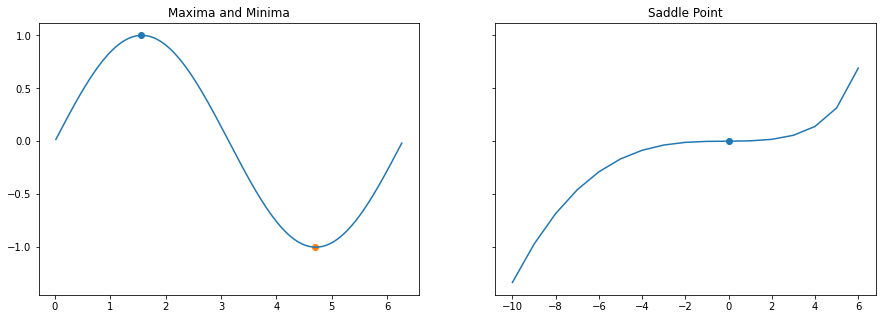### Multiple Minimas

When there are more than one minimas or saddle points - often the optimization alogrithms fails. Escaping from a saddle point is a deep topic of research and this article just introduces what exactly they are.

x1 = np.arange(-20, 20, 1)
y = np.arange(-20, 20, 1)
plt.figure(figsize=(15, 5))
y1 = x1**3 * np.cos(x1) - x1 * np.sin(x1)
plt.plot(x1, y1)
points = [[-19, -6784], [-16, 3927.19], [-13, -2000], [0, 0], [10, -900], [13, 2000], [16, -4000]]
[plt.scatter(pt, pt) for pt in points]

plt.text(-19, -6784, 'Presumably Global Minima', style ='italic',
fontsize = 12, color ="green")
plt.text(-13, -2000, 'Local Minima', style ='italic',
fontsize = 12, color ="green")
plt.text(-16, 3296, 'Local Maxima', style ='italic',
fontsize = 12, color ="green")
plt.text(0, 0, 'Saddle Point', style ='italic',
fontsize = 12, color ="green")
plt.show()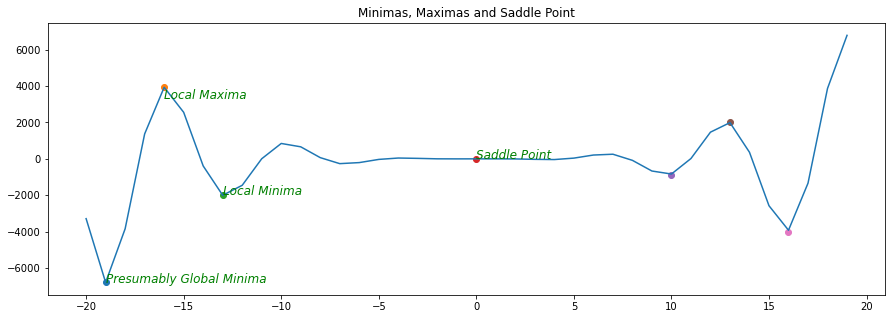### Multivariate Functions

Until now we saw univariate functions where the outcome is decided by a single variable. However machine learning systems are multi-dimensional and we often come across complex cost function functions. Let us say, we have a function with multiple variables $f(x_1, x_2, x_3, \cdots, x_n)$ i.e. the features of the dataset then the derivative of that function becomes quite difficult. This is addressed through partial derivatives as follows…

Let us say we have 3 variables $x, y, z$ in our dataset then our differentiable function is $$f(x, y, z)$$ $$then$$ $$f'(x, y, z) = \frac{\partial f }{\partial x} + \frac{\partial f }{\partial y} + \frac{\partial f }{\partial z}$$ i.e. We can differentiate anything with respect to anything. We represent these partial derivatives in matrix form and it is called as Jacobian Matrix. $$J = \left [ \frac{\partial f }{\partial x}, \frac{\partial f }{\partial y}, \frac{\partial f }{\partial z} \right]$$ Jacobian is a row vector. We can generalize this as follows $$f(x_1, x_2, x_3, \cdots, x_n)$$ $$then$$ $$J(x_1, \cdots, x_n) = \nabla_x f(x) = \left [ \frac{\partial f }{\partial x_1}, \frac{\partial f }{\partial x_2}, \frac{\partial f }{\partial x_3}, \cdots, \frac{\partial f }{\partial x_n} \right] \tag{5. Jacobian Matrix}$$ Further, Jacobian is a vector that we can calculate for each and every location on the space. The steeper the slope, the greater the magnitude of the Jacobian.

Critical points are the locations where every element of the gradient is equal to zero,

$$\nabla_x f(x) = 0 = \begin{cases} \frac{\partial f}{\partial x_1} = 0 \ \frac{\partial f}{\partial x_2} = 0 \ \vdots \ \frac{\partial f}{\partial x_n} = 0 \end{cases} \tag{6. Critical Point}$$

In this section, we shall explore regression problem using Ordinary Least Square methods. OLS are the best methods for residual analysis, where it penalises when the errors are large. This section has an overview to linear regression and in depth implementation of non-linear regression for a Gaussian Distribution.

### Linear Least Squares

We shall start our Linear Regression from the equation of a line $y=mx+c$ as follows

$$y = y(x;a_i) = mx_i + c$$ then the residual of the fitted line is $r_i$ and the overall efficacy of the fitted line is $\chi^2$. i.e. $$\chi^2 = \sum_{i=0}^n r_i^2 = \sum_{i=0}^n (y_i - mx_i - c)^2 \tag{7. Chi-Squared loss}$$ where there are $n$ observersions.

### Jacobian of Chi-Squared Loss

Our goal is to identify the right value for the gradient(slope) $m$ and the intercept $c$. Let us do partial derivative of the loss function wrt $m, c$ to make a Jacobian matrix.

from $equ.7$, differentiate wrt $m$ $$\frac{\partial \chi^2}{\partial m} = \sum_i 2 * (y_i - mx_i - c)^{2-1} * -x_i$$ $$i.e$$ $$\frac{\partial \chi^2}{\partial m} = -2\sum_i x_i(y_i - mx_i - c)$$

from $equ.7$, differentiate wrt $c$ $$\frac{\partial \chi^2}{\partial c} = \sum_i 2 * (y_i - mx_i - c)^{2-1} * -1$$ $$i.e$$ $$\frac{\partial \chi^2}{\partial c} = -2\sum_i (y_i - mx_i - c)$$

$$hence \ the \ jacobian \ matrix \ is$$ $$\nabla \chi^2 = \left( \begin{array}{cc} \frac{\partial{\chi^2}}{\partial{m}}, \ \frac{\partial \chi^2}{\partial c} \end{array} \right) = \left( \begin{array}{cc} -2\sum_i x_i(y_i - mx_i - c), \ -2\sum_i (y_i - mx_i - c) \end{array} \right) \tag{8. Jacobian for Linear Regression}$$

Refer, a complete implementation of Linear Lease Squares is demonstrated using Tensorflow Gradient Tapes in this post

### Non Linear Least Squares

We shall examine a non linear function of Gaussian distribution with 4 fitting parameters $(\sigma, \mu, I, b)$ and build a Jacobian matrix. $$\Large y(x; \sigma, \mu, I, b) = b + \frac {I}{\sigma\sqrt{2\pi}} e^{ \left( - \frac{(x - \mu)^2}{2\sigma^2} \right) }$$

def gaussian(x, mu, sigma, I=1, b=0):
return b + I * np.exp(-(x-mu)**2/(2*sigma**2)) / (np.sqrt(2*np.pi) * sigma)


### Jacobian of Gaussian Function

then it’s Jacobian Matrix is $$\Large \nabla \chi^2 = \left[\begin{array}{cc} \frac{\partial \chi^2}{\partial \sigma} \ \frac{\partial \chi^2}{\partial \mu} \ \frac{\partial \chi^2}{\partial I} \ \frac{\partial \chi^2}{\partial b} \end{array}\right]$$

The following derivative is done by hand,

$$\frac{\partial \chi^2}{\partial \sigma} = \frac {I}{\sigma\sqrt{2\pi}} e^{ \left( - \frac{(x - \mu)^2}{2\sigma^2} \right) } * \left(\frac{(x-\mu)^2}{\sigma^3} - \frac{1}{\sigma} \right)$$

$$\frac{\partial \chi^2}{\partial \mu} = \frac{I}{\sqrt{2\pi}} \frac{(x - \mu)}{\sigma^3} e^{ \left( - \frac{(x - \mu)^2}{2\sigma^2} \right) }$$

$$\frac{\partial \chi^2}{\partial I} = \frac {1}{\sigma\sqrt{2\pi}} e^{ \left( - \frac{(x - \mu)^2}{2\sigma^2} \right) }$$

$$\frac{\partial \chi^2}{\partial b} = 1$$

and the Jacobian matrix for the Gaussian Distribution as follows

$$\nabla \chi^2 = \left[\begin{array}{cc} \frac{\partial \chi^2}{\partial \sigma} \ \frac{\partial \chi^2}{\partial \mu} \ \frac{\partial \chi^2}{\partial I} \ \frac{\partial \chi^2}{\partial b} \end{array}\right] = \left[\begin{array}{cc} \frac {I}{\sigma\sqrt{2\pi}} e^{ \left( - \frac{(x - \mu)^2}{2\sigma^2} \right) } * \left(\frac{(x-\mu)^2}{\sigma^3} - \frac{1}{\sigma} \right), \ \frac{I}{\sqrt{2\pi}} \frac{(x - \mu)}{\sigma^3} e^{ \left( - \frac{(x - \mu)^2}{2\sigma^2} \right) }, \ \frac {1}{\sigma\sqrt{2\pi}} e^{ \left( - \frac{(x - \mu)^2}{2\sigma^2} \right) }, \ 1 \end{array}\right] \tag{9. Jacobian of Gaussian Distribution}$$

### Build the Partial Derivatives

There are four fitting parameters $(\sigma, \mu, I, b)$, We shall build four methods that accomplish partial derivatives wrt them

• $\frac{\partial \chi^2}{\partial \sigma}$ - dchi2_dsigma
• $\frac{\partial \chi^2}{\partial \mu}$ - dchi2_dmu
• $\frac{\partial \chi^2}{\partial I}$ - dchi2_dI
• $\frac{\partial \chi^2}{\partial b}$ - dchi2_db
# ∂𝜒2/∂𝜎
def dchi2_dsigma(x, mu, sigma, I=1, b=0):
return gaussian(x, mu, sigma, I, b) * (((x-mu)**2 / sigma**3) - (1/sigma))

# ∂𝜒2/∂𝜇
def dchi2_dmu(x, mu, sigma, I=1, b=0):
return gaussian(x, mu, sigma, I, b) * ((x-mu) / sigma**2)

# ∂𝜒2/∂𝐼
def dchi2_dI(x, mu, sigma, I=1, b=0):
return np.exp(-(x-mu)**2/(2*sigma**2)) / (np.sqrt(2*np.pi) * sigma)

# ∂𝜒2/∂𝑏
def dchi2_db(x, mu, sigma, I=1, b=0):
return 1


### Ordinary Differential Equation - Step Function

Single step of length $(\sigma, \mu, I, b)$ is multiplied to solve the differential equation.

def step_fn(x, y, mu, sig, I=1, b=0, aggression=2000):
J = np.array([
-2 * (y - gaussian(x, mu, sig)) @ dchi2_dmu(x, mu, sigma),
-2 * (y - gaussian(x, mu, sig)) @ dchi2_dsigma(x, mu, sigma),
-2 * (y - gaussian(x, mu, sig)) @ dchi2_dI(x, mu, sigma),
-2 * (y - gaussian(x, mu, sig)) * dchi2_db(x, mu, sigma)
])

step = -J * aggression
return step

def ode(x, y_init, mu_pred, sigma_pred, I_pred, b_pred):
preds = np.array([[mu_pred, sigma_pred, I_pred, b_pred]])
for i in range(100):
dmu, dsigma, dI, db = step_fn(x, y_init, mu_pred, sigma_pred, I_pred, b_pred, 2000)
mu_pred += dmu
sigma_pred += dsigma
I_pred += dI
b_pred += db
preds = np.append(preds, [[mu_pred, sigma_pred, I_pred, b_pred]], axis=0)

return preds, mu_pred, sigma_pred, I_pred, b_pred


## Simulations

Let us build helper functions to create and visualize the distributions and convergence.

def plot_distributions(x, y, y_init):
fig, axs = plt.subplots(1, 2, figsize=(20, 5), sharey=True)
axs.plot(x, y)
axs.set_title("Actual Distribution")
axs.plot(x, y_init, color="green")
axs.set_title("Initial Distribution for Prediction")

plt.show()

def create_distribution(x, mu_init = 25, sigma_init = 3):
mu = np.mean(x)
sigma = np.std(x)
y = gaussian(x, mu, sigma)
y_init = gaussian(x, mu_init, sigma_init)

return x, y, mu, sigma, y_init, mu_init, sigma_init

def compare_distributions(x, y, y_pred):
plt.figure(figsize=(10, 5))
plt.ylim([0, 0.02])
plt.plot(x, y, label="Actual Distribution")
plt.plot(x, y_pred, label="Predicted Distribution")
plt.legend()
plt.show()

def convergence_plot(x, y, preds):
plt.figure(figsize=(11, 6))

for i in np.arange(0, len(preds)):
if(i % 10 == 0):
y1 = gaussian(x, preds[i], preds[i])
plt.plot(x, y1, label=f"{i}th iteration, $\mu$={preds[i]} $\sigma$={preds[i]}", linestyle='dashdot')
plt.legend()
plt.plot(x, y, color="green", linewidth=4, label=f"Actual Distribution $\mu$={mu} $\sigma$={sigma}")
plt.legend()
plt.title("Convergence Plot")
plt.show()


### Actual and Initial Distributions

We are creating two distribution, 1 is the actual data and the other one initialized with a random mean($\mu_{actual}$) and random standard deviation($\sigma_{actual}$). Our goal is to used the data of the first distribution and predicted ($\mu_{pred}, \sigma_{pred}$).
Let us compare the actual and initial distributions visually.

x, y, mu, sigma, y_init, mu_init, sigma_init = create_distribution(np.arange(0, 100, 1))
plot_distributions(x , y, y_init)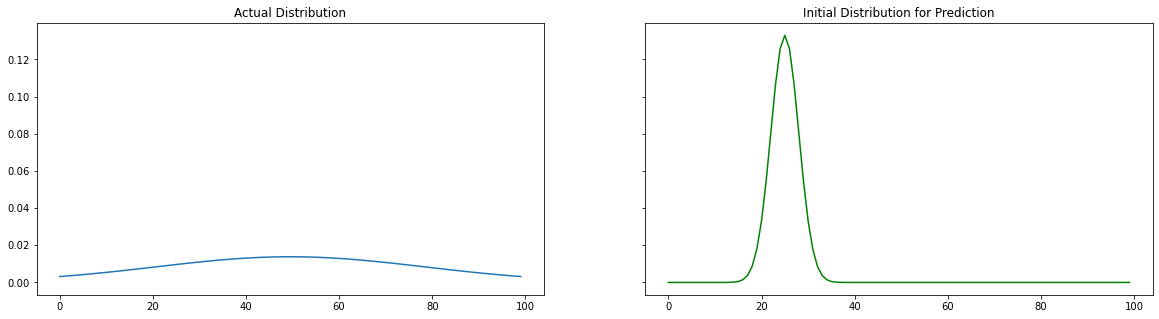### Predicted Disribution

Using our ODE iterator function, we have calculated ($\mu_{pred}, \sigma_{pred}$). Using new mean and standard deviation, $y_{pred}$ calculated and visualization aligns with very minimal error.

I_init = 1
b_init = 0
preds, mu_pred, sigma_pred, I_pred, b_pred = ode(x, y, mu_init, sigma_init, I_init, b_init)
y_pred = gaussian(x, mu_pred, sigma_pred)
compare_distributions(x, y, y_pred)

<ipython-input-5-af1d68394805>:2: VisibleDeprecationWarning: Creating an ndarray from ragged nested sequences (which is a list-or-tuple of lists-or-tuples-or ndarrays with different lengths or shapes) is deprecated. If you meant to do this, you must specify 'dtype=object' when creating the ndarray
J = np.array([
<__array_function__ internals>:5: VisibleDeprecationWarning: Creating an ndarray from ragged nested sequences (which is a list-or-tuple of lists-or-tuples-or ndarrays with different lengths or shapes) is deprecated. If you meant to do this, you must specify 'dtype=object' when creating the ndarray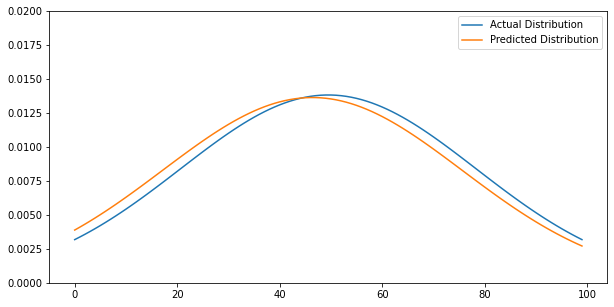### Convergence

Using convergence plot, examine the step wise convergence to reach the optimal point.

convergence_plot(x, y, preds)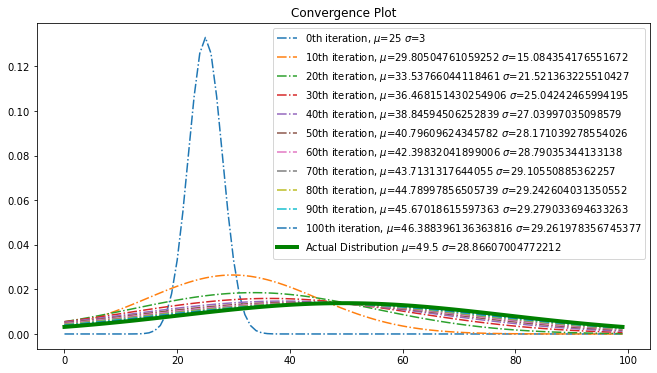## Inference

The most significant aspect of this post is not using any sophisticated packages to calculate the gradient descent. This is more a proof of the efficacy of Jacobian matrix in optimization challenges. This post is an incomplete one, I planned to add the following topics in the futre posts

• Hessian Matrix for learning rate determination and measure of curvature
• Eigen values, vectors and spaces: One of my favorite topics
• Taylors series, Newton Raphson method and Lagrange Multipliers